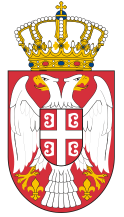Dokument se učitava

# Signals and SystemsCourse code: 140217 | 6 ECTS credits

Basic information
Level of Studies: Undergraduate applied studies
Year of Study: 1
Semester: 2
Requirements:
Goal: The objective of the course is that students earn knowledge on continuous and discrete signals and systems, both in time and frequency domain.
Outcome: Knowledge of basic signals, types of signals and operations over signals. Application of transformations for switching from time to complex and frequency domain. Knowledge of linear, time invariant systems. Knowledge that is needed for all later subjects where the signals and systems are studied and also their analysis, modeling and processing.
Contents of the course
Theoretical instruction:
1. Introduction to the theory of signals and systems. Basic characteristics and types of signals and systems.
2. Operations over continuous signals.
3. Overview and properties of continuous systems.
4. Characteristics of continuous linear stationary systems.
5. Differential equations and their application. Fundamentals of differential equations.
6. Elementary discrete signals.
7. Overview and properties of discrete systems.
8. Laplace transform. Characteristics of Laplace transform. Inverse Laplace transformation.
9. System transfer function and one-sided Laplace transform. Impulse and step response.
10. Decomposition of the transfer function to partial fractions. Algebra of the transfer function.
11. Periodic continuous signals and Fourier series.
12. Aperiodic continuous signals and Fourier transform.
13. Convergence of the Fourier transform. Amplitude and phase of the signal spectrum.
14. Characteristics and application of the Fourier transform. The sampling theorem.
15. Colloquium.
Practical instruction (Problem solving sessions/Lab work/Practical training):
1. It is performed in a hardware laboratory with the active participation of students. Students independently or with the help of teachers solve the tasks based on the set exercises in the Manual.
Textbooks and References
1. T. Petrović, A. Rakić, Signals and systems, DEXIN, Belgrade, 2005.
2. A. Marjanović, P. Tadić, V. Papić, Signals and systems-collection of solved problems, Academic Thought, Beograd, 2013.
3. B. Kovačević, Ž. Đurović, Automatic control systems- collection of solved problems, Science – Belgrade, 1995.
Number of active classes (weekly)
Lectures: 2
Practical classes: 3
Other types of classes: 0
Grading (maximum number of points: 100)
Pre-exam obligations
Points
activities during lectures
5
activities on practial excersises
15
seminary work
0
colloquium
40
Final exam
Points
Written exam
40
Oral exam
0© 2019 School of Electrical and Computer Engineering of Applied Studies, Belgrade
Vojvode Stepe 283, office@viser.edu.rs, +381 11 2471 099